Courses

# PERT And CPM Inventory Control - MCQ Test 1

## 20 Questions MCQ Test Mock Test Series - Mechanical Engineering (ME) for GATE 2020 | PERT And CPM Inventory Control - MCQ Test 1

Description
This mock test of PERT And CPM Inventory Control - MCQ Test 1 for Mechanical Engineering helps you for every Mechanical Engineering entrance exam. This contains 20 Multiple Choice Questions for Mechanical Engineering PERT And CPM Inventory Control - MCQ Test 1 (mcq) to study with solutions a complete question bank. The solved questions answers in this PERT And CPM Inventory Control - MCQ Test 1 quiz give you a good mix of easy questions and tough questions. Mechanical Engineering students definitely take this PERT And CPM Inventory Control - MCQ Test 1 exercise for a better result in the exam. You can find other PERT And CPM Inventory Control - MCQ Test 1 extra questions, long questions & short questions for Mechanical Engineering on EduRev as well by searching above.
QUESTION: 1

Solution:
QUESTION: 2

Solution:
QUESTION: 3

### Micromotion study involves following number of fundamental hand motions

Solution:
QUESTION: 4

There are two products P and Q with the following characteristics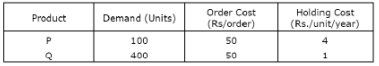The economic order quantity (EOQ) of products P and Q will be in the ratio

Solution: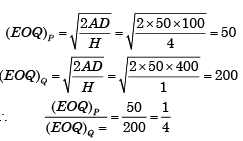QUESTION: 5

A dummy activity is used in PERT network to describe

Solution:
QUESTION: 6

In inventory planning, extra inventory is unnecessarily carried to the end of the planning period when, using one of the following lot size decision policies:

Solution:
QUESTION: 7

In PERT, the distribution of activity times is assumed to be:

Solution:
QUESTION: 8

Market demand for springs is 8,00,000 per annum. A company purchases these springs in lots and sells them. The cost of making a purchase order is Rs.1,200. The cost of storage of springs is Rs.120 per stored piece per annum. The economic order quantity is:

Solution: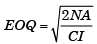Where, N = 8, 00, 000; A = 1200 Rsm; CI = 120 Rs/stored piece/annum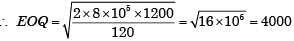QUESTION: 9

The expected time (te) of a PERT activity in terms of optimistic time (to), pessimistic time (tp) and most likely time (t1) is given by:

Solution:
QUESTION: 10

An item can be purchased for Rs 100. The ordering cost is Rs. 200 and the inventory carrying cost is 10% of the item cost annum. If the annual demand is 4000 units, then economic order quantity (in units) is:

Solution: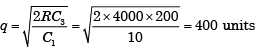QUESTION: 11

Consider a PERT network for a project involving six tasks (a to f)The expected completion time of the project is:

Solution:

Critical path = a – c – e – f
= 30 + 60 + 45 + 20
= 155 days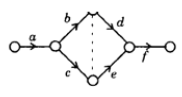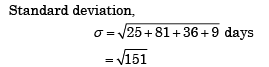QUESTION: 12

Consider a PERT network for a project involving six tasks (a to f)The standard deviation of the critical path of the project is:

Solution:
QUESTION: 13

If the demand for an item is doubled and the ordering cost halved, the economic order quantity

Solution: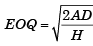where, A = Ordering cost; D = Demand; h = Unit holding cost

QUESTION: 14

Consider the following PERT network:

The optimistic time, most likely time and pessimistic time of all the activities are given in the table below: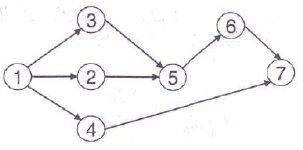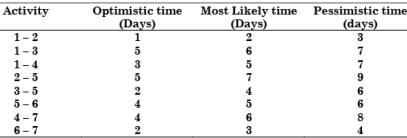The critical path duration of the network (in days) is:

Solution:
QUESTION: 15

Consider the following PERT network:

The optimistic time, most likely time and pessimistic time of all the activities are given in the table below:The standard deviation of the critical path is:

Solution:
QUESTION: 16

Annual demand for window frames is 10000. Each frame costs Rs. 200 and ordering cost is Rs. 300 per order. Inventory holding cost is Rs. 40 per frame per year. The supplier is willing to offer 2% discount if the order quantity is 1000 or more, and 4% if order quantity is 2000 or more. If the total cost is to be minimized, the retailer should

Solution: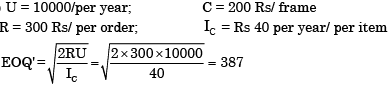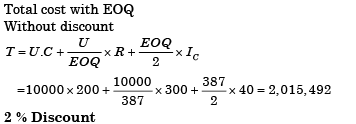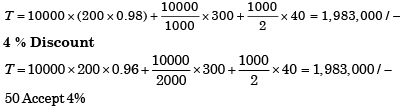QUESTION: 17

For the network below, the objective is to find the length of the shortest path from node P to node G. Let dij be the length of directed arc from node i to node j. Let sj be the l ength of the shortest path from P to node j. Which of the following equations can be used to find sG?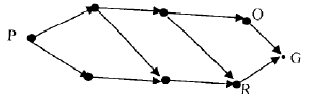Solution:
QUESTION: 18

A company has an annual demand of 1000 units, ordering cost of Rs. 100/ order and carrying cost of Rs. 100/unit-year. If the stock-out costs are estimated to be nearly Rs. 400 each time the company runs out-of-stock, then safety stock justified by the carrying cost will be:

Solution:

Given: D = 1000;   Ordering cost, A = Rs. 100/order
Holding cost, H = Rs. 100/unit-year; Stock out cost, S = Rs. 400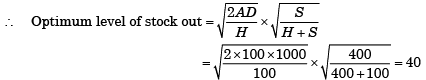QUESTION: 19

A Project consists of activities A to M shown in the net in the following figure with the duration of the activities marked in days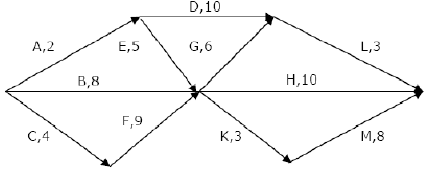The project can be completed:

Solution:

Project completed = Activity C + Activity F + Activity K +  Activity M
= 4 + 9 + 3 + 8 = 24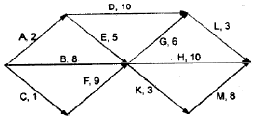QUESTION: 20

The maximum level of inventory of an item is 100 and it is 100 and it is achieved with infinite replenishment rate. The inventory becomes zero over one and half month due to consumption at a uniform rate. This cycle continues throughout the year. Ordering cost is Rs. 100 per order and inventory carrying cost is Rs. 10 per item per month. Annual cost (in Rs.) of the plan, neglecting material cost, is:

Solution: